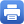Columbia Home
Complex Analysis and Riemann Surfaces

This course is taken in sequence, part 1 in the fall, and part 2 in the spring.

### COMPLEX ANALYSIS AND RIEMANN SURFACES I

Local theory of holomorphic functions
The Riemann surface of $w^2=z(z-1)(z-\lambda)$
Construction of holomorphic forms and meromorphic forms with prescribed poles
Abel’s theorem
The Jacobi inversion theorem

Function theory on tori:

• The point of view of Weierstrass
• The point of view of Jacobi: $\theta$ functions
• The point of view of partial differential equations

Line bundles, connections, metrics, curvature, first Chern class
The Riemann-Roch theorem, applications
The Riemann-Roch theorem as an index theorem
A heat equation proof of the Riemann-Roch theorem
Function theory on Riemann Surfaces of higher genus:
Theta functions, the theta divisor, the prime form
Theta characteristics and spinors

### COMPLEX ANALYSIS AND RIEMANN SURFACES II

Holomorphic vector bundles on complex manifolds
Connections and curvature, characteristic classes and Chern-Weil theory
The Uniformization theorem
The Ricci curvature and the Calabi conjecture:
Statement and complete proof; estimates for the complex Monge-Ampere equation
Hermitian-Einstein metrics and Mumford stability
The Donaldson-Uhlenbeck-Yau theorem
The proof by Uhlenbeck and Yau

Depending on the year, the last part of II (Hermitian-Einstein metrics, etc) may be replaced by a treatment of the $\bar\partial$ equation, as follows:

• Bochner-Kodaira formulas
• H\”ormander’s estimates for the $\bar\partial$ equation
• Singular metrics and multiplier ideal sheaves
• The Kodaira imbedding theorem
• The Ohsawa-Takegoshi extension theoremPrint this page